3d Printing: Cube and Octahedron
The Cube and the Octahedron are dual polyhedra

In this page we are going to build the cube and the octahedron using a 3d printer. Then we are going to see that these two polyhedra are dual polyhedra.

The Cube

The cube is a very well known polyhedron.To model these vertices I used OpenSCAD, a free wonderful program.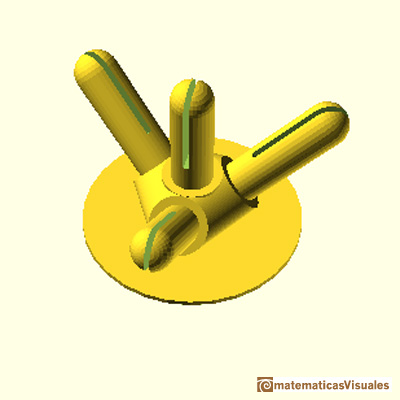We can count its faces (C), edges (A) and vertices (V):

To calculate the circumradius (the radius of a circumsphere touching each of the cube's vertices) we can start calculating the diagonal d of one face: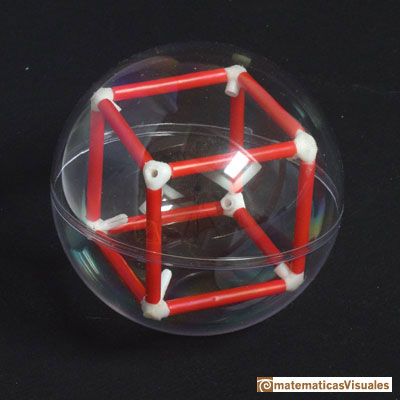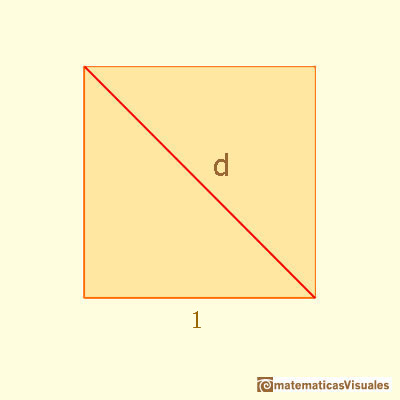Then the diagonal of the cube (the space diagonal) D is:This section of the cube is related with the DinA size of paper: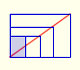There is a standarization of the size of the paper that is called DIN A. Successive paper sizes in the series A1, A2, A3, A4, and so forth, are defined by halving the preceding paper size along the larger dimension.

One diagonal of this rectangle: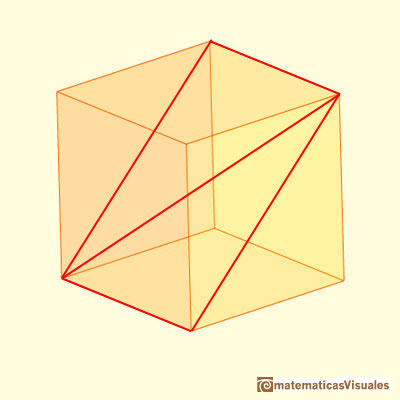You can calculate D as a basic application of the Pythagorean Theorem:The inradius of a cube is (the radius of the insphere or inscribed sphere that is tangent to each of the cube's faces):

The Octahedron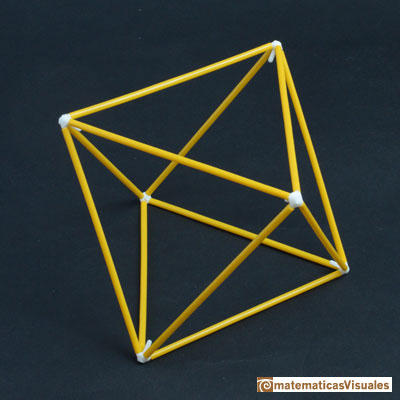The volume of an octahedron is four times the volume of a tetrahedron. It is easy to calculate and then we can get the volume of a tetrahedron.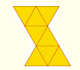The first drawing of a plane net of a regular octahedron was published by Dürer in his book 'Underweysung der Messung' ('Four Books of Measurement'), published in 1525 .

As always, I used OpenSCAD, a free wonderful program, to model these vertices: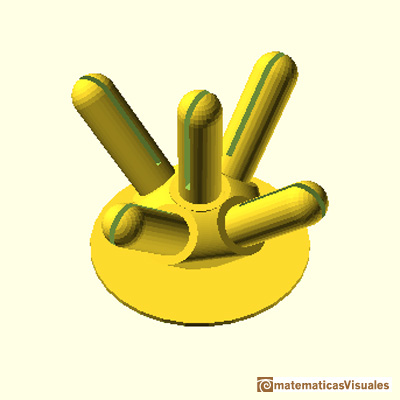We can count its faces (C), edges (A) and vertices (V):

Now we are going to calculate le circumradius of an octahedron.We can see the height of these two pyramides as the diagonal of a square.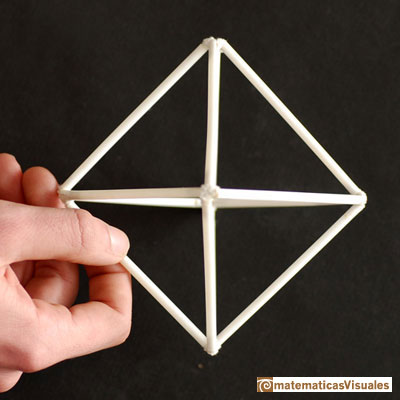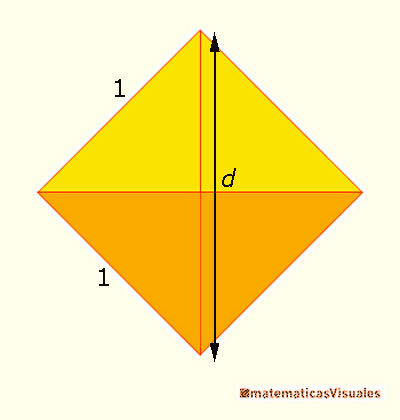The diagonal of a square of edge length 1 is:

The circumradius of an octahedron of edge length a is:

The inradius of an octahedron is: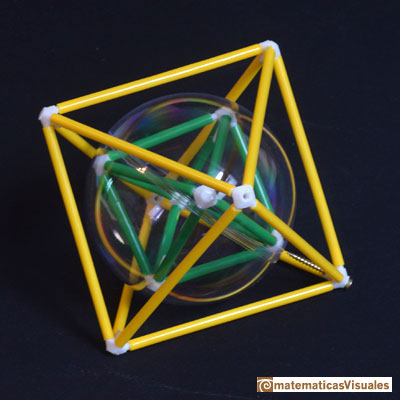The Cube and the Octahedron are dual polyhedra

The vertices of the cube correspond with the faces of the octahedron and vice versa. And both have the same number of edges.

Then we say that the cube and the octahedron are dual polyhedra.

One way to contruct a dual polyhedron of a regular polyhedron is to choose the center of the faces and connect each point with the points of its neighboring faces.

We are going to do this with the cube and the octahedron.

An Octahedron inside a Cube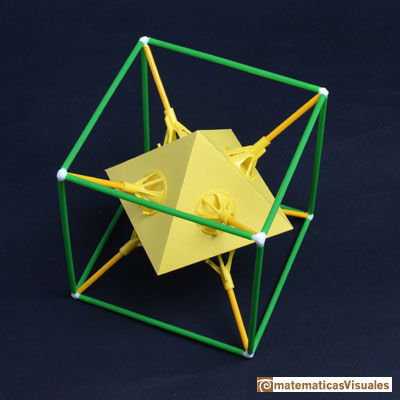The octahedron has edge length l and the edge length of the cube is L. Then the circumradius of the octahedron must be equal to the inradius of the cube: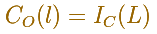A Cube inside an Octahedron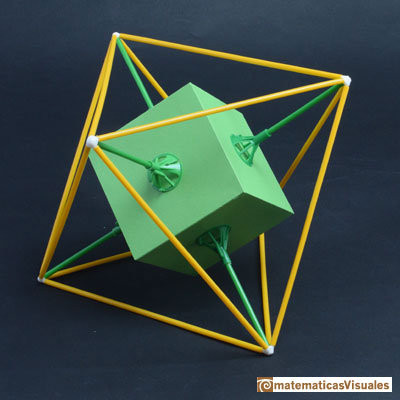The cube has edge length l and the edge length of the octahedron is L. Then the circumradius of the cube must be equal to the inradius of the octahedron: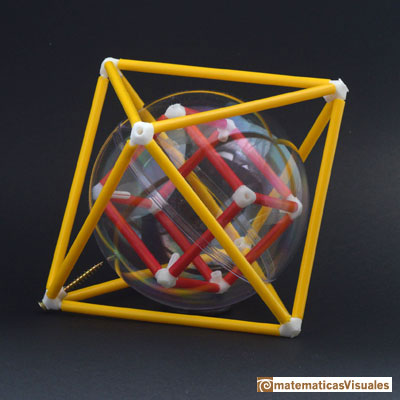There is an easy way to see the relation between the edge lengths of the inscribed cube l and the octahedron L. See this picture: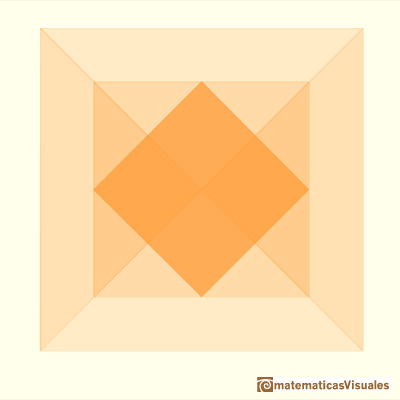The four edges of the square on the middle pass through four barycenters of four faces of the big octahedron.REFERENCES

OpenSCAD a free wonderful program to model shapes in three dimensions.
Magnus Wenninger - 'Polyhedron Models', Cambridge University Press.
Hugo Steinhaus - Mathematical Snapshots - Oxford University Press - Third Edition (p. 197)
Peter R. Cromwell - 'Polyhedra', Cambridge University Press, 1999.
H.Martin Cundy and A.P. Rollet, 'Mathematical Models', Oxford University Press, Second Edition, 1961 (p. 87).
W.W. Rouse Ball and H.S.M. Coxeter - 'Matematical Recreations & Essays', The MacMillan Company, 1947.There is a standarization of the size of the paper that is called DIN A. Successive paper sizes in the series A1, A2, A3, A4, and so forth, are defined by halving the preceding paper size along the larger dimension.The volume of an octahedron is four times the volume of a tetrahedron. It is easy to calculate and then we can get the volume of a tetrahedron.The first drawing of a plane net of a regular octahedron was published by Dürer in his book 'Underweysung der Messung' ('Four Books of Measurement'), published in 1525 .Simple technique to build polyhedra gluing discs made of cardboard or paper.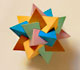Using cardboard you can build beautiful polyhedra cutting polygons and glue them toghether. This is a very simple and effective technique. You can download several templates. Then print, cut and glue: very easy!Material for a session about polyhedra (Zaragoza, 9th May 2014). Simple techniques to build polyhedra like the tetrahedron, octahedron, the cuboctahedron and the rhombic dodecahedron. We can build a box that is a rhombic dodecahedron.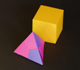Material for a session about polyhedra (Zaragoza, 7th November 2014). We study the octahedron and the tetrahedron and their volumes. The truncated octahedron helps us to this task. We build a cubic box with cardboard and an origami tetrahedron.Material for a session about polyhedra (Zaragoza, 23rd Octuber 2015) . Building a cube with cardboard and an origami octahedron.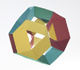Material for a session about polyhedra (Zaragoza, 21st October 2016). Instructions to build several geometric bodies.Italian designer Bruno Munari conceived 'Acona Biconbi' as a work of sculpture. It is also a beautiful game to play with colors and shapes.Microarquitectura is a construction game developed by Sara San Gregorio. You can play and build a lot of structures modelled on polyhedra.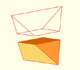Special sections of a tetrahedron are rectangles (and even squares). We can calculate the area of these cross-sections.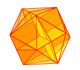The twelve vertices of an icosahedron lie in three golden rectangles. Then we can calculate the volume of an icosahedronSome properties of this platonic solid and how it is related to the golden ratio. Constructing dodecahedra using different techniques.